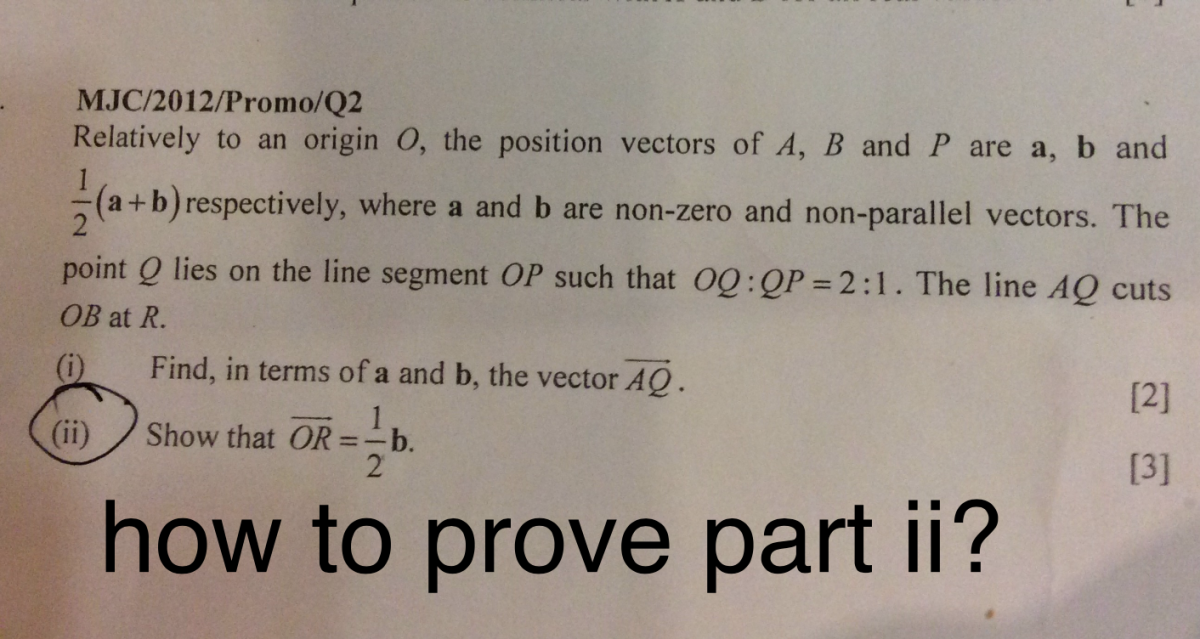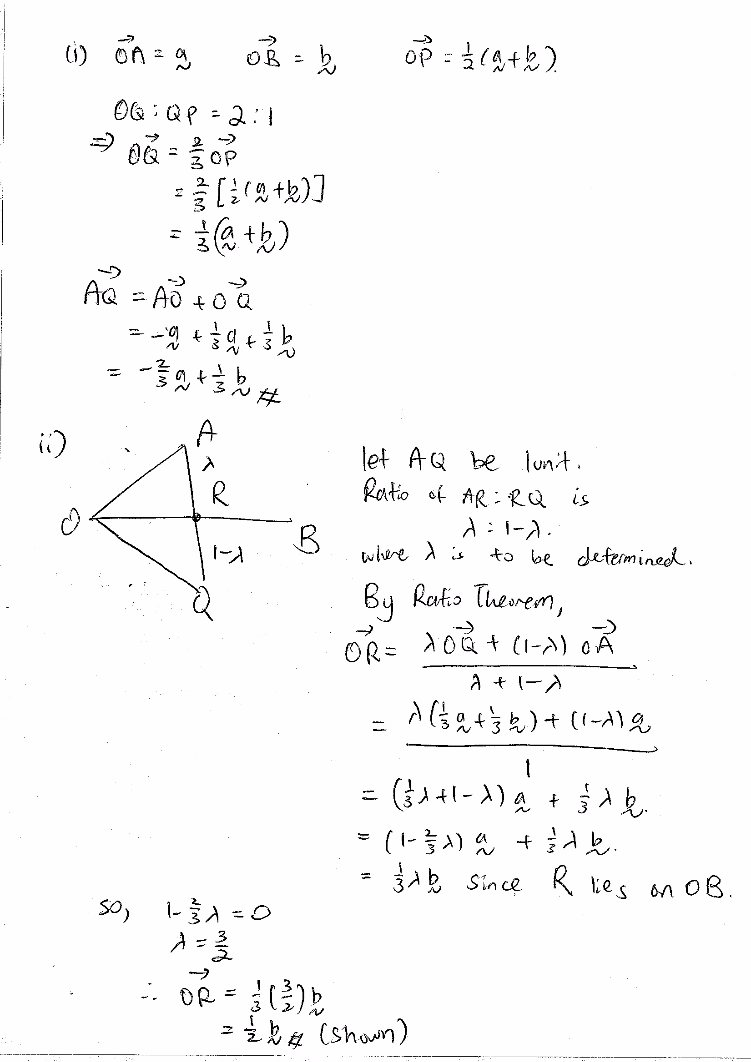# QuestionSource: mjc promo question 2012 paper 2 q 20 Replies 1 Like

AQ=OQ-OA

=2/3(1/2a+1/2b)-a

=-2/3a+1/3b

OR = kOB =kb(as OR is along OB, where k is a constant to be found ——-(1)

AR = pAQ =p(-2/3a+1/3b),where p is a constant to be found

OR-OA=p(1/3b)-p(2/3a)

OR=a+p(1/3b)-p(2/3a)

=p(1/3b)+(1-2p/3a)———-(2)

(1)=(2)

k=p(1/3),

1-2p/3=0

so, p=3/2,k=1/2

Sub it back to (1),

conclude that OR=1/2b

0 Replies 1 Like